#### MAT-HS.A-REI.02

 MAT-HS Targeted Standards(A) Concept: Algebra(REI) Domain: Reasoning with Equations and InequalitiesCluster: Understand solving equations as a process of reasoning and explain the reasoning MAT-HS.A-REI.02 Solve simple rational and radical equations in one variable, and give examples showing how extraneous solutions may arise.

## Student Learning Targets:

• I can
• I can

• I can
• I can

### Skills (Performance) Targets

• I can solve simple rational and radical equations in one variable and provide examples of how extraneous solutions arise.

• I can
• I can

## Alg II Solve Radical Equations Proficiency Scale

 Score Description Sample Activity 4.0 (advanced) In addition to Score 3.0, the student demonstrates in-depth inferences and applications regarding more complex material that go beyond end of instruction expectations. Solve the following equation: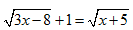3.5 In addition to Score 3.0 performance, the student demonstrates in-depth inferences and applications regarding the more complex content with partial success. 3.0 (proficient) The student can: solve a radical equation and identify an extraneous solution. provide examples of how extraneous solutions to a radical equation arise. The student exhibits no major errors or omissions. Solve the following equations: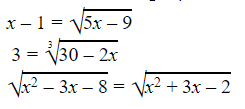2.5 The student demonstrates no major errors or omissions regarding the simpler details and processes (Score 2.0 content) and partial knowledge of the more complex ideas and processes (Score 3.0 content). 2.0 (progressing) There are no major errors or omissions regarding the simpler details and processes as the student can: recognize and recall specific terminology such as: radical radicand index inverse operations extraneous solution identify an extraneous solution to a radical equation when given possible solutions.      However, the student exhibits major errors or omissions regarding the more complex ideas and processes. Solve the following equations: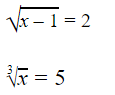1.5 The student demonstrates partial knowledge of the simpler details and processes (Score 2.0 content) but exhibits major errors or omissions regarding the more complex ideas and procedures (Score 3.0 content). 1.0 (beginning) With help, the student demonstrates a partial understanding of some of the simpler details and processes (Score 2.0 content) and some of the more complex ideas and processes (Score 3.0 content). 0.5 With help, the student demonstrates a partial understanding of some of the simpler details and processes (Score 2.0 content) but not the more complex ideas and processes (Score 3.0 content). 0.0 Even with help, the student demonstrates no understanding or skill.

## Alg II Solve Rational Equations Proficiency Scale

Score   Description Sample Activity

4.0

In addition to Score 3.0, the student demonstrates in-depth inferences and applications regarding more complex material that go beyond end of instruction expectations.

 Describe the error in the following work: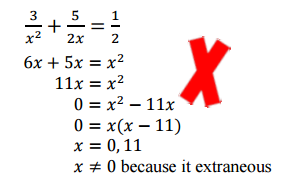3.5 In addition to Score 3.0 performance, the student demonstrates in-depth inferences and applications regarding the more complex content with partial success.

3.0

(proficient)

The student can:
• solve a rational equation and recognize an extraneous solution.
The student exhibits no major errors or omissions.

Solve the following rational equation for r  and check for extraneous solutions: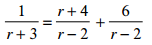2.5 The student demonstrates no major errors or omissions regarding the simpler details and processes (Score 2.0 content) and partial knowledge of the more complex ideas and processes (Score 3.0 content).

2.0

(progressing)

There are no major errors or omissions regarding the simpler details and processes as the student can:
• recognize and recall specific terminology such as:
• rational equation
• denominator
• extraneous solution
• solve simple rational equations.
However, the student exhibits major errors or omissions regarding the more complex ideas and processes.

Solve the following rational equation for n: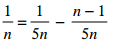1.5 The student demonstrates partial knowledge of the simpler details and processes (Score 2.0 content) but exhibits major errors or omissions regarding the more complex ideas and procedures (Score 3.0 content).

1.0

(beginning)

With help, the student demonstrates a partial understanding of some of the simpler details and processes (Score 2.0 content) and some of the more complex ideas and processes (Score 3.0 content).
0.5 With help, the student demonstrates a partial understanding of some of the simpler details and processes (Score 2.0 content) but not the more complex ideas and processes (Score 3.0 content).
0.0 Even with help, the student demonstrates no understanding or skill.

## Resources

 Web Vocab